College Physics : Continuity Equation

Example Questions

Example Question #1 : Continuity Equation

Fluid flows into a pipe with a diameter of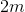at a rate of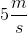. If the other end of the pipe has a cross sectional area of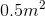, what is the speed of the fluid as it exits the pipe?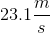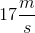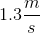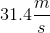Explanation:

This question tests the concept of the continuity equation which stipulates that at steady state the volume flowing into one end of a pipe must be exactly equal to the volume flowing out of the other end. That is,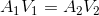. Note that in this case, we are given the inlet diameter and not cross-sectional area. Thus, we must also find the inlet's cross-sectional area by using the formula for the area of a circle.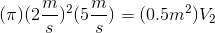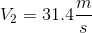Example Question #64 : College Physics

If the radius of a pipe with flowing liquid is decreased by a factor of, how does the velocity of the fluid need to change in order to maintain a constant flow rate?

Increase by a factor ofIncrease by a factor ofDecrease by a factor ofDcrease by a factor ofIncrease by a factor ofExplanation:

To see how a change of radius affects the velocity of a moving fluid, we'll need to take the continuity equation into account.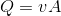Even though the radius is not shown in the above expression, remember that area is related to radius.Or put another way: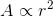When substituted into the continuity equation: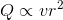As the above proportionality shows, if the radius were to decrease by a factor of, this would cause the flow rate to decrease by a factor of. To counteract this drop in flow rate, the velocity term would need to increase by a factor of.

All College Physics Resources